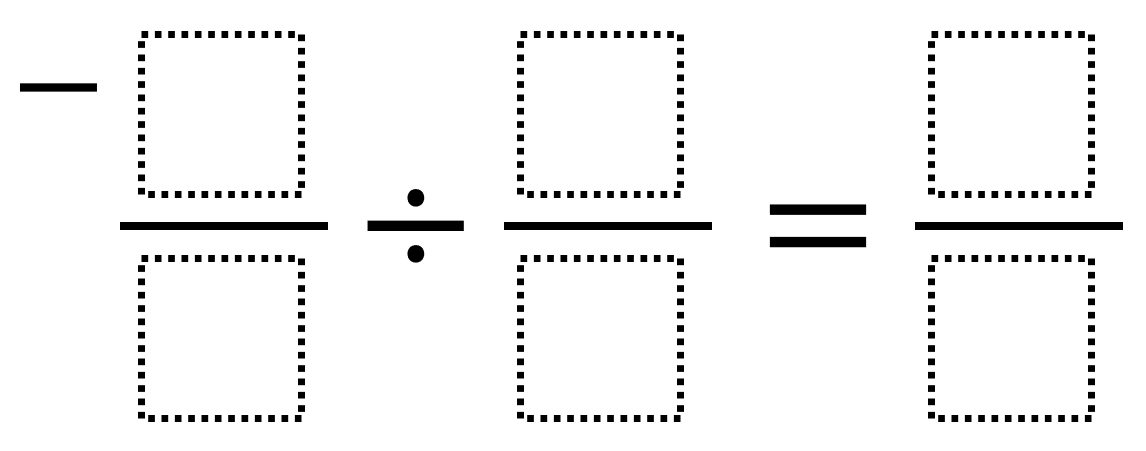# Multiplying And Dividing Rational Numbers 1

Directions: Using the integers -9 to 9 at most one time each, fill in the boxes to create two equations. You may reuse all the integers for each equation.### Hint

Which digits would be impossible to use? Why?